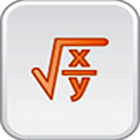Mathematics Formula Reference

All Android applications categories

All Android games categories# Mathematics Formula Reference

by: 1.2K 8.2

8.2 Users
rating

## Screenshots

Description

Math Formula Reference is a simple application that helps you access a vast collection of math formulas, with thousands of
mathematical equations.
This app can help you as a quick reference (cheat sheet) so you can access math formulas whenever and wherever you want.
This math formulary app is one of the most comprehensive of its kind.

Math Formula Reference covers the following topics:

1. Number Sets
2. Algebra
3. Geometry (Plane and Spatial)
4. Trigonometry
5. Matrices and Determinants
6. Vectors

This app can be moved to the SD Card.
Please, feel free to report bugs related to the content and this app.

[Usage Instructions]
1) Open Math Formula Reference.
2) Select the math topic you want to browse.
3) Select the subtopic you want to view.
4) View the formulas related to the subtopic you selected.

Tags: formulas matemātikā , this free app is ad supported and may contain ads in the notification tray and/or home screen , fórmula matematica referencia , 免費快速數學視頻 , mathematical equations , mathematics formula , mathematics equation commented photo , math formula view , formules mathématiques pro

## Users review

from 1.204 reviews

"Great"

8.2